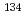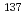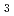Compton-to-peak analysis is a method for selecting suitable coefficients to convert count rates measured with in situ gamma ray spectrometry to radioactivity concentrations ofCs &Cs in the environment. The Compton-to-peak method is based on the count rate ratio of the spectral regions containing Compton scattered gamma rays to that with the primaryCs &Cs photopeaks. This is known as the Compton-to-peak ratio (RCP). RCP changes as a function of the vertical distribution ofCs &Cs within the ground. Inferring this distribution enables the selection of appropriate count rate to activity concentration conversion coefficients. In this study, the PHITS Monte Carlo radiation transport code was used to simulate the dependency of RCP on different vertical distributions ofCs &Cs within the ground. A model was created of a LaBr(Ce) detector used in drone helicopter aerial surveys in Fukushima Prefecture. The model was verified by comparing simulated gamma ray spectra to measurements from test sources. Simulations were performed for the infinite half-space geometry to calculate the dependency of RCP on the mass depth distribution (exponential or uniform) ofCs &Cs within the ground, and on the altitude of the detector above the ground. The calculations suggest that the sensitivity of the Compton-to-peak method is greatest for the initial period following nuclear fallout whenCs &Cs are located close to the ground surface, and for aerial surveys conducted at low altitudes. This is because the relative differences calculated between RCP with respect to changes in the mass depth distribution were largest for these two cases. Data on the measurement height above and on theCs &Cs activity ratio is necessary for applying the Compton-to-peak method to determine the distribution and radioactivity concentration ofCs &Cs in the ground.